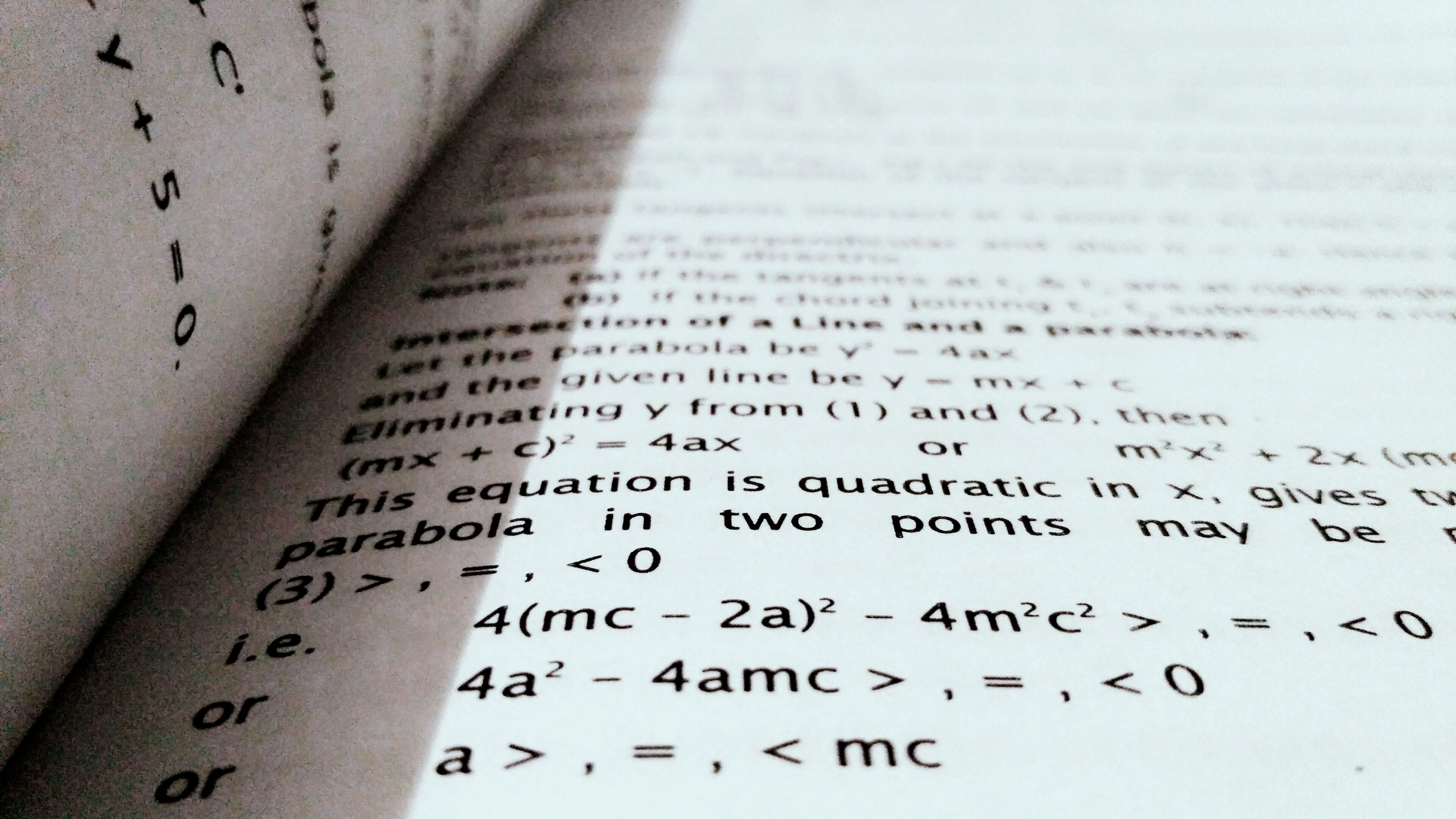Conceptual Approach - One Dimensional Motion - Part - III, Class XI - Physics - Student's Corner

# Student's Corner

Website is dedicated for students. You will get a large amount of study material. Students who cannot afford quality education will get high benefits. All the knowledge and details of competitive examinations will published time to time. Students with Physics and Mathematics background will be highly beneficial.

## Subscribe Us

In the beginning there was nothing, which exploded.

# Conceptual Approach - One Dimensional Motion - Part - II, Class XI - Physics

## What is average acceleration?

The average acceleration of a particle during a time interval is defined as the ratio of change in velocity to the time interval. Acceleration is a vector quantity. Therefore it has magnitude as well as direction. The SI unit of acceleration is m/s2.

Note 1: The acceleration of a moving particle may be positive or negative. The negative acceleration is sometimes referred as retardation or deceleration.

Note 2: We don’t use vector symbol for acceleration in one dimensional motion. Positive or negative sign is sufficient.

## What is Instantaneous acceleration?

It is defined as time rate change of velocity. In simple words, similar to velocity, acceleration at a particular instant is called as instantaneous acceleration.

## Important:

All the topics covered in previous blogs were for introduction. And none of these will help you to crack any examination. If you dealing in physics, then you must know the calculus. In next blog we will see calculus.

Ask us @ Quora : Advanced Tech World

Join the Group : Advanced Tech WorldMathematics